# HBSE 7th Class Maths Solutions Chapter 5 Lines and Angles Ex 5.2

Haryana State Board HBSE 7th Class Maths Solutions Chapter 5 Lines and Angles Ex 5.2 Textbook Exercise Questions and Answers.

## Haryana Board 7th Class Maths Solutions Chapter 5 Lines and Angles Exercise 5.2

Question 1.
State the property that is used in each of the following statements?
(i) If a // b, then ∠1 = ∠5.
(ii) If ∠4 – ∠6, then a //b.
(iii) If ∠4 + ∠5 = 180°, then a // b.
Solution: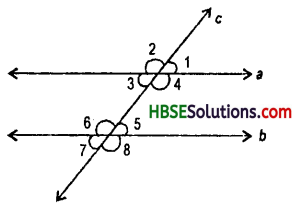If two lines are intersected by a transversal and if we have any of the following three conditions.
(a) The angles of any pair of corresponding angles are equal.
(b) The angles of any pair of alternate angles are equal.
(c) The sum of the interior angles on the same side of the transversal is 180°, then the lines are parallel.
(i) In the above figure, if a || b, then ∠1 = ∠5 = corresponding angles.
(ii) If ∠4 = ∠8 – corresponding angles, hence a || b.
(iii) If ∠4 + ∠5 = 180° = supplementary, hence a||b.Question 2.
In the given figure, identify :
(i) The pairs of corresponding angles.
(ii) The pairs of alternate interior angles.
(iii) The pairs of interior angles on the same side of the transversal.
(iv) The vertically opposite angles.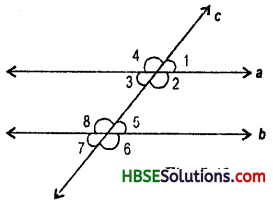Solution:
(i) ∠1 and ∠5, ∠4 and ∠8, ∠3 and ∠7, ∠2 and ∠6.
(ii) ∠3 and ∠5, ∠2 and ∠8
(iii) ∠2 and ∠5, ∠3 and ∠8
(iv) ∠1 and ∠3, ∠4 and ∠2, ∠8 and ∠6, ∠5 and ∠7.

Question 3.
In the adjoining figure, p || q. Find the unknown angles.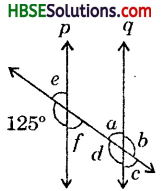Solution:
∠e + ∠125° = 180°(Linearpair)
∴ ∠e = 180°-125° = 55°
∠e = ∠f = 55° (vertically opposite angles)
∠d = 125° (corresponding angles)
∠d = ∠b =125° (vertically opposite angles)
∠e = ∠a = 55° (corresponding angles)
∠a = ∠c = 55° (vertically opposite angles)
i.e., a = 55°, b = 125°, c = 55°, d = 125°, e = 55° and f = 55°.Question 4.
Find the value of x in each of the following figures if l || m.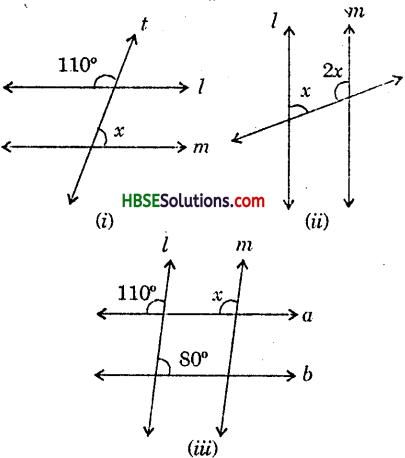Solution:
(i) ∠x = ∠x = (alternate angles)
New ∠x + ∠100° = 180° (Linear pair)
=> Lx = 180°—110°
∴ x = 70°.(Fig.i)
(ii) x + 2x = 1800 (supplementary angles)
3x = 180°
x = $$\frac{180}{3}$$
∴ x = 60°.
x = 100 (Corresponding angles)
or, y + 80° = 180° (Supplemcnatary angles)
∴ y = 100°
s = y = 100° (alternate angles)
(Fig. iii)

Question 5.
In the given figure, the arms of two angles are parallel. If ∠DGC = 70° then find s
(i) ∠DGC
(ii) ∠DEF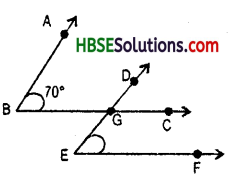Solution:
∠ABC = ∠DGC = corresponding angles
= 70°
∴ ∠DGC = 70°
∠DGC = ∠DEF = 70°
= corresponding angles
hence, (i) ∠DGC = 70°
(ii) ∠DEF = 70°Question 6.
In the given figures below, decide whether l is parallel to m ?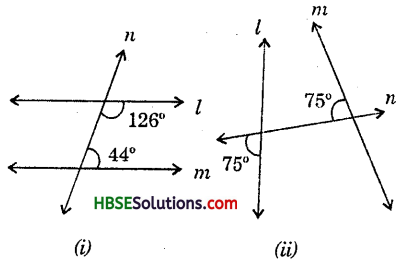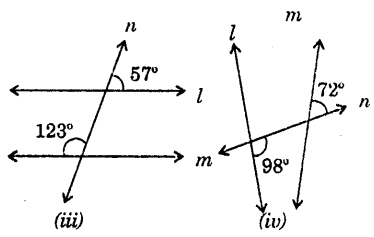Solution:
(i) ∠126° + ∠44° = 170° ≠ 180° (Corresponding angles)
Hence l is not parallel to m.
(ii) l is not parallel to m because alternate angles are not equal.
(iii) ∠57° + x = 180° (Linear pair)
∴ x = 180° -57° = 123°
Now x = 123° = alternate angle
Hence l || m because alternate angles are equal.
(iv) l is not parallel to m because alternate
angles are not equal. .